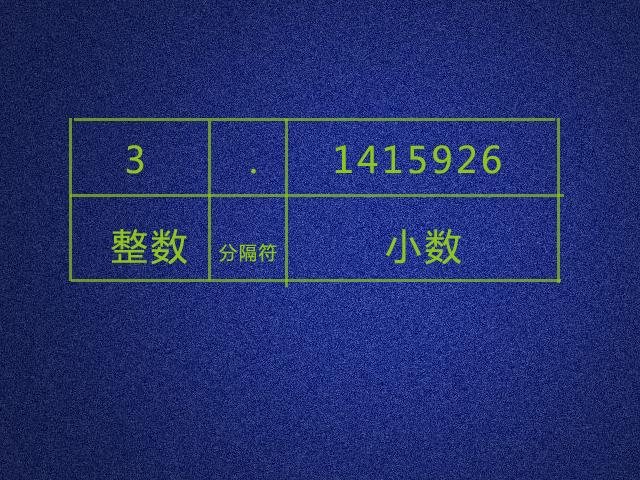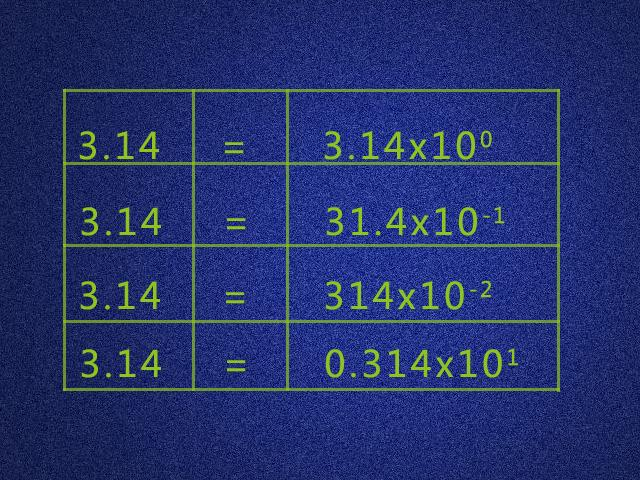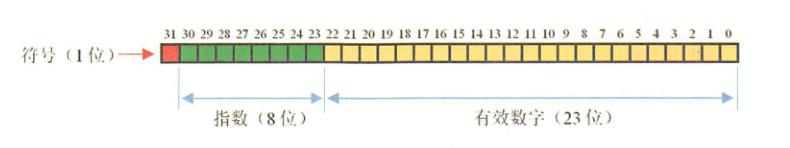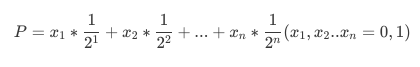# Double为什么会失真？## 前言

0.1-0.0=0.1
0.2-0.0=0.2
0.2-0.1=0.1
0.3-0.0=0.3
0.3-0.1=0.19999999999999998
0.3-0.2=0.09999999999999998
0.4-0.0=0.4
0.4-0.1=0.30000000000000004
0.4-0.2=0.2
0.4-0.3=0.10000000000000003
0.5-0.0=0.5
0.5-0.1=0.4
0.5-0.2=0.3
0.5-0.3=0.2
0.5-0.4=0.09999999999999998
0.6-0.0=0.6
0.6-0.1=0.5
0.6-0.2=0.39999999999999997
0.6-0.3=0.3
0.6-0.4=0.19999999999999996
0.6-0.5=0.09999999999999998
0.7-0.0=0.7
0.7-0.1=0.6
0.7-0.2=0.49999999999999994
0.7-0.3=0.39999999999999997
0.7-0.4=0.29999999999999993
0.7-0.5=0.19999999999999996
0.7-0.6=0.09999999999999998
0.8-0.0=0.8
0.8-0.1=0.7000000000000001
0.8-0.2=0.6000000000000001
0.8-0.3=0.5
0.8-0.4=0.4
0.8-0.5=0.30000000000000004
0.8-0.6=0.20000000000000007
0.8-0.7=0.10000000000000009
0.9-0.0=0.9
0.9-0.1=0.8
0.9-0.2=0.7
0.9-0.3=0.6000000000000001
0.9-0.4=0.5
0.9-0.5=0.4
0.9-0.6=0.30000000000000004
0.9-0.7=0.20000000000000007
0.9-0.8=0.09999999999999998

## 一、 什么是浮点数？

### 1、小数### 2、小数为什么会被称为浮点数## 二、浮点数在内存中是如何存储的？float 符号位(1bit) 指数(8 bit) 尾数(23 bit)

double 符号位(1bit) 指数(11 bit) 尾数(52 bit)

float在内存中占8位，由于阶码实际存储的是指数的移码，假设指数的真值是e,阶码为E，则有E=e+(2^n-1 -1)。其中 2^n-1 -1是IEEE754标准规定的指数偏移量，根据这个公式我们可以得到 2^8 -1=127。于是，float的指数范围为-128 +127，而double的指数范围为-1024 +1023。其中负指数决定了浮点数所能表达的绝对值最小的非零数；而正指数决定了浮点数所能表达的绝对值最大的数，也即决定了浮点数的取值范围。

float的范围为-2^128 ~ +2^127，也即-3.40E+38 ~ +3.40E+38；

double的范围为-2^1024 ~ +2^1023，也即-1.79E+308 ~ +1.79E+308

0 000 0000 - 0 111 1111

1 000 0000 - 1 111 1111

## 三、浮点数的进制转换

### 1、十进制转二进制

• 整数部分：整数除以2，得到一个商和余数，得到的商继续除以2并得到一个商和一个余数，继续除以2操作直至商为0，上述操作得到一系列余数，从最后一个余数开始直至第一个余数，这一系列0\1即为转换后的二进制数。

• 小数部分：乘以2，然后取出整数部分，将剩下的小数部分继续乘以2，然后再取整数部分，一直取到小数部分为零为止。如果永远不为零，则按要求保留足够位数的小数，最后一位做0舍1入。将取出的整数顺序排列。true
false
true

20转换后变为 10100
0.3 要转换二进制，需要乘2, 乘完之后 取整数部分，然后用乘的结果减去整数部分， 然后 接着乘2, 直至最后没有小数或者小数出现循环, 即乘完.

0.3 * 2 = 0.6 (0)
0.6 * 2 = 1.2 (1)
0.2 * 2 = 0.4 (0)
0.4 * 2 = 0.8 (0)
0.8 * 2 = 1.6 (1)

0.3 = 0.010011001…1001

### 2、二进制的科学记数法表示

20.3 = 10100.010011001…1001 (二进制)=1.01000100110011E10…..（十进制科学计数）=1.01000100110011E100…..(二进制科学计数)

### 4、机器ε

1.000……0000（52个0） × 2^0

1.000……0001 × 2^0

2^-52 ≈ 2.220446049250313e-16。所以它就是双精度浮点数的机器ε。

CSDN